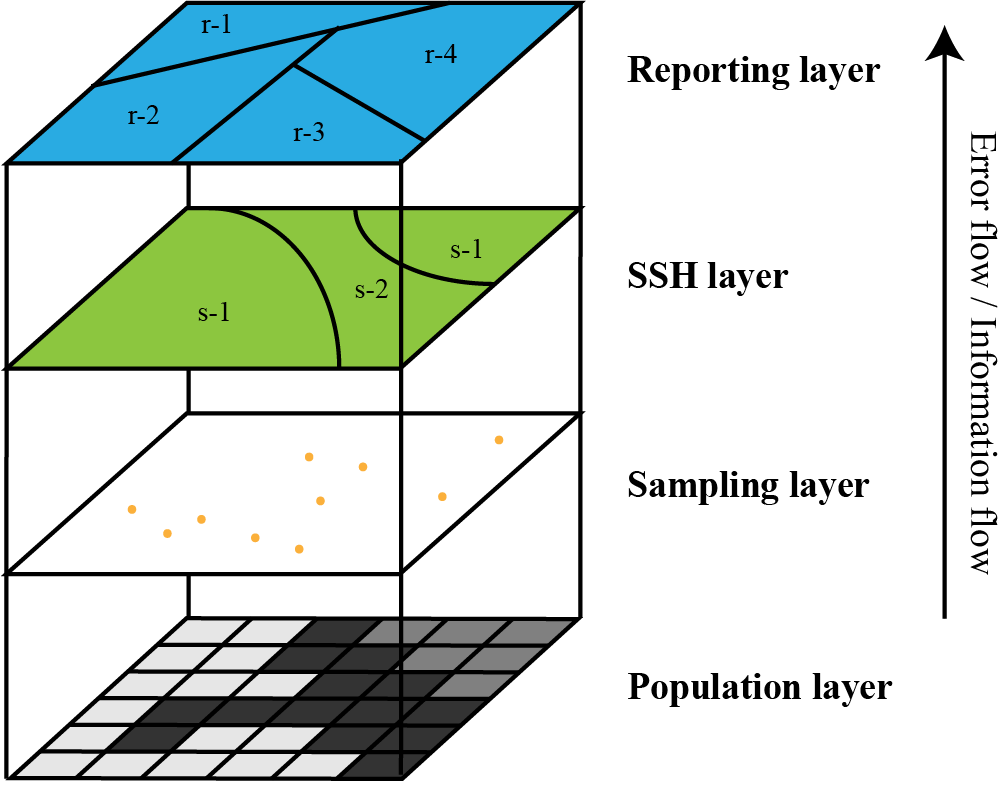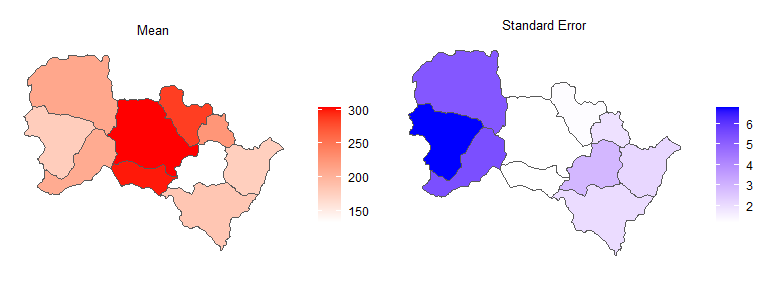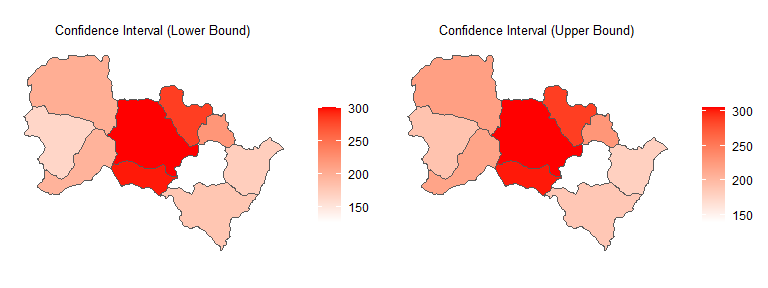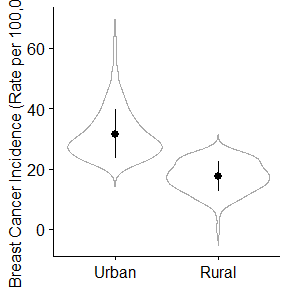# A Tutorial for the sandwichr R Package

#### 2022-02-08

The sandwichr package performs spatial interpolation1 based on the spatial stratified heterogeneity (SSH) theory2. This model requires three inputs: sampling, SSH, and reporting layers. The goal of this model is to estimate the mean value of the sampling attribute and its standard error for each reporting unit leveraging the known distribution of the population in the SSH layer. Since this model does not rely on the spatial dependence of the population, when the spatial dependence is weak, Sandwich is still applicable as long as one properly stratifies the geospatial surface. Figure 1 is an overview of the model structure.Figure 1. Structure of the SSH-based spatial interpolation model.

To use the sandwichr package, first install it from source code (only do this once).

# Install the sandwichr package
# install.packages("sandwichr")

Then load the installed package into your environment using library(). Remember to load the package every time you use it.

# Import the sandwichr package and other packages
library("sandwichr")
library(ggplot2)
library(ggpubr)
library(dplyr)

Here,we present two case studies to demonstrate the functionality of this package.

## Human population in Heshun County, China

The first case study interpolates the human population density in Heshun County, China based on a sample of 167 units. A zonation consists of 5 spatially contiguous strata is used as a candidate SSH layer. The administrative division of 10 townships in Heshun County is used as the reporting layer. The sampling, SSH, and reporting layers are all in the shapefile format.

To successfully run the model, the load.data.shp function is used to prepare the shapefiles of sampling, SSH, and reporting layers into a list of sf (simple feature; see Simple Features for R for references) objects for model input. You can specify the directory and names of your input files. Note that these input files should locate in the same di

# Input data from shapefiles
hs.sampling.name <- system.file("extdata", "hs.sampling.shapefile.shp",
package="sandwichr")
hs.ssh.name <- system.file("extdata", "hs.ssh.shapefile.shp",
package="sandwichr")
hs.reporting.name <- system.file("extdata", "hs.reporting.shapefile.shp",
package="sandwichr")

ssh.file=hs.ssh.name,
reporting.file=hs.reporting.name)

# Sampling
head(hs.data[])
## Simple feature collection with 6 features and 4 fields
## Geometry type: POINT
## Dimension:     XYZ
## Bounding box:  xmin: 156491.4 ymin: 4126601 xmax: 171510.5 ymax: 4153214
## z_range:       zmin: 1320.701 zmax: 1500
## Projected CRS: WGS 84 / UTM zone 50N
## # A tibble: 6 x 5
##   CODE  Population     x     y                      geometry
##   <chr>      <dbl> <dbl> <dbl>                   <POINT [m]>
## 1 02033         31  113.  37.5     Z (156491.4 4153214 1500)
## 2 01007         23  113.  37.2 Z (165240.2 4126601 1320.701)
## 3 01008         38  113.  37.2   Z (166538 4127516 1354.123)
## 4 01009         89  113.  37.3 Z (169540.8 4129381 1370.255)
## 5 01010         22  113.  37.3     Z (170685.2 4130558 1400)
## 6 01011        129  113.  37.3     Z (171510.5 4131899 1400)
class(hs.data[])
##  "sf"         "tbl_df"     "tbl"        "data.frame"
attributes(hs.data[])
## $names ##  "CODE" "Population" "x" "y" "geometry" ## ##$row.names
##      1   2   3   4   5   6   7   8   9  10  11  12  13  14  15  16  17  18
##    19  20  21  22  23  24  25  26  27  28  29  30  31  32  33  34  35  36
##    37  38  39  40  41  42  43  44  45  46  47  48  49  50  51  52  53  54
##    55  56  57  58  59  60  61  62  63  64  65  66  67  68  69  70  71  72
##    73  74  75  76  77  78  79  80  81  82  83  84  85  86  87  88  89  90
##    91  92  93  94  95  96  97  98  99 100 101 102 103 104 105 106 107 108
##  109 110 111 112 113 114 115 116 117 118 119 120 121 122 123 124 125 126
##  127 128 129 130 131 132 133 134 135 136 137 138 139 140 141 142 143 144
##  145 146 147 148 149 150 151 152 153 154 155 156 157 158 159 160 161 162
##  163 164 165 166 167
##
## $class ##  "sf" "tbl_df" "tbl" "data.frame" ## ##$sf_column
##  "geometry"
##
## $agr ## CODE Population x y ## <NA> <NA> <NA> <NA> ## Levels: constant aggregate identity # SSH head(hs.data[]) ## Simple feature collection with 5 features and 3 fields ## Geometry type: POLYGON ## Dimension: XY ## Bounding box: xmin: 151114.4 ymin: 4106864 xmax: 228423.6 ymax: 4165211 ## Projected CRS: WGS 84 / UTM zone 50N ## # A tibble: 5 x 4 ## STR Area STR_1 geometry ## <int> <dbl> <chr> <POLYGON [m]> ## 1 1 681. 01 ((174393.7 4131444, 174755.7 4136086, 166016.2 4129531, 161~ ## 2 2 445. 02 ((211986.7 4145078, 191141.7 4123426, 191052.7 4123478, 190~ ## 3 3 288. 03 ((198113.2 4115589, 201902.7 4118894, 204447.9 4121855, 211~ ## 4 4 346. 04 ((222674.5 4139326, 219575.5 4133270, 215318.4 4129568, 211~ ## 5 5 427. 05 ((172634.5 4164622, 171285.3 4160901, 169971.9 4159759, 173~ class(hs.data[]) ##  "sf" "tbl_df" "tbl" "data.frame" attributes(hs.data[]) ##$names
##  "STR"      "Area"     "STR_1"    "geometry"
##
## $row.names ##  1 2 3 4 5 ## ##$class
##  "sf"         "tbl_df"     "tbl"        "data.frame"
##
## $sf_column ##  "geometry" ## ##$agr
##   STR  Area STR_1
##  <NA>  <NA>  <NA>
## Levels: constant aggregate identity
# Reporting
head(hs.data[])
## Simple feature collection with 6 features and 2 fields
## Geometry type: POLYGON
## Dimension:     XY
## Bounding box:  xmin: 151114.4 ymin: 4123480 xmax: 214095.3 ymax: 4165211
## Projected CRS: WGS 84 / UTM zone 50N
## # A tibble: 6 x 3
##   CODE   Area                                                           geometry
##   <chr> <dbl>                                                      <POLYGON [m]>
## 1 02    427.  ((153690.1 4149820, 153647.4 4149999, 153508.5 4150431, 153309.4 ~
## 2 06    143.  ((191225.5 4151326, 191387.6 4151395, 191542.2 4151533, 191625.6 ~
## 3 03    373.  ((178892 4151731, 179098.2 4151712, 179205.5 4151708, 179415.6 41~
## 4 05    252.  ((172768 4143250, 172542.9 4143207, 172248.9 4143115, 171995.3 41~
## 5 10     73.7 ((207615.6 4147664, 207785.4 4147473, 207928.5 4147388, 208079.1 ~
## 6 01    186.  ((172768 4143250, 173154.8 4143172, 173615.2 4143071, 173995.6 41~
class(hs.data[])
##  "sf"         "tbl_df"     "tbl"        "data.frame"
attributes(hs.data[])
## $names ##  "CODE" "Area" "geometry" ## ##$row.names
##    1  2  3  4  5  6  7  8  9 10
##
## $class ##  "sf" "tbl_df" "tbl" "data.frame" ## ##$sf_column
##  "geometry"
##
## $agr ## CODE Area ## <NA> <NA> ## Levels: constant aggregate identity ### Selection of the SSH layer(s) The accuracy of SSH-based spatial interpolation is determined by the SSH layer. For an ideal SSH layer, values of the target attribute are expected to be homogeneous within each stratum and differ between the strata. To determine a proper SSH layer for the Sandwich model, the geographical detector model3 is applied to quantify the SSH of the target attribute with regard to the candidate stratification(s). For the purpose of demonstration, we input hs.ssh2.shapefile as another candidate SSH layer. library(sf) library(tools) # Input another candidate SSH layer for demonstration hs.ssh2.name <- system.file("extdata", "hs.ssh2.shapefile.shp", package="sandwichr") hs.ssh2 <- read_sf(dsn=dirname(hs.ssh2.name), layer=file_path_sans_ext(basename(hs.ssh2.name))) First, we combine the sampling and the candidate SSH layers to a single data frame. # Prepare the SSH layer(s) for evaluation hs.join <- ssh.data.shp(object=hs.data[], ssh.lyr=hs.data[], ssh.id="STR_1") hs.join <- ssh.data.shp(object=hs.join, ssh.lyr=hs.ssh2, ssh.id="STR_2") head(hs.join) ## Simple feature collection with 6 features and 6 fields ## Geometry type: POINT ## Dimension: XYZ ## Bounding box: xmin: 156491.4 ymin: 4126601 xmax: 171510.5 ymax: 4153214 ## z_range: zmin: 1320.701 zmax: 1500 ## Projected CRS: WGS 84 / UTM zone 50N ## # A tibble: 6 x 7 ## CODE Population x y geometry STR_1 STR_2 ## <chr> <dbl> <dbl> <dbl> <POINT [m]> <chr> <chr> ## 1 02033 31 113. 37.5 Z (156491.4 4153214 1500) 05 11 ## 2 01007 23 113. 37.2 Z (165240.2 4126601 1320.701) 05 02 ## 3 01008 38 113. 37.2 Z (166538 4127516 1354.123) 05 02 ## 4 01009 89 113. 37.3 Z (169540.8 4129381 1370.255) 05 02 ## 5 01010 22 113. 37.3 Z (170685.2 4130558 1400) 05 02 ## 6 01011 129 113. 37.3 Z (171510.5 4131899 1400) 05 02 The factor detector q-statistic in the geographical detector model is applied through the ssh.test function to measure the SSH of the sampling data in terms of different stratifications. This function takes a data frame generated from the ssh.data.shp (or ssh.data.txt, which will be introduced later) function (argument object), where the field names of the sampling attribute (argument y) and the strata ID(s) (argument x) need to be specified. In this example, the output of ssh.test implies that the original candidate SSH layer (q = .91) has a higher determinant power over the attribute (population density) compared to hs.ssh2 (q = .39). Therefore, compared to hs.ssh2, it will be more reasonable to select the original one as the SSH layer for subsequent interpolation. # Calculate the geographical detector q-statistic ssh.test(object=hs.join, y="Population", x=c("STR_1", "STR_2"), test="factor") ## [] ## q-statistic p-value ## STR_1 0.5841001 3.386389e-10 ## ## [] ## q-statistic p-value ## STR_2 0.2540372 8.683782e-06 The ssh.test function implements the interaction detector in the geographical detector model by specifying test="interaction". The result of our example shows that the combined q-statistic of the original candidate SSH layer and hs.ssh2 (q = .92) is approximately the same as that of using the original one alone (q = .91). Therefore, we can either optimize the SSH layer by combining two SSH layers or simply use the original one. For the purpose of simplicity, we will use the original one alone as the SSH layer in the subsequent modeling. # Calculate the interaction detector ssh.test(object=hs.join, y="Population", x=c("STR_1", "STR_2"), test="interaction") ## [,1] [,2] [,3] ## [1,] "STR_1" "STR_2" "0.620092311481712" ## [2,] "STR_1" "STR_1" "0.58410012034806" ## [3,] "STR_2" "STR_2" "0.254037208669126" ### Running the Sandwich model The SSH-based spatial interpolation is performed using the sandwich.model function, which outputs the mean value of the sampling attribute and its standard error for each reporting unit. # Perform the SSH based spatial interpolation hs.sw <- sandwich.model(object=hs.data, sampling.attr="Population", type="shp") head(hs.sw$object)
## Simple feature collection with 6 features and 5 fields
## Geometry type: POLYGON
## Dimension:     XY
## Bounding box:  xmin: 151114.4 ymin: 4123480 xmax: 214095.3 ymax: 4165211
## Projected CRS: WGS 84 / UTM zone 50N
## # A tibble: 6 x 6
##   CODE   Area                                         geometry  mean    se    df
##   <chr> <dbl>                                    <POLYGON [m]> <dbl> <dbl> <dbl>
## 1 02    427.  ((153690.1 4149820, 153647.4 4149999, 153508.5 ~  211.  5.28    91
## 2 06    143.  ((191225.5 4151326, 191387.6 4151395, 191542.2 ~  287.  1.28    18
## 3 03    373.  ((178892 4151731, 179098.2 4151712, 179205.5 41~  304.  1.21    90
## 4 05    252.  ((172768 4143250, 172542.9 4143207, 172248.9 41~  178.  6.80    91
## 5 10     73.7 ((207615.6 4147664, 207785.4 4147473, 207928.5 ~  225.  1.94    40
## 6 01    186.  ((172768 4143250, 173154.8 4143172, 173615.2 41~  207.  5.46    91
summary(hs.sw)
##  "Sample size:  167"
##  "Number of SSH strata:  5"
##  "Number of reporting units:  10"
##       mean             se                 geometry
##  Min.   :133.7   Min.   :1.214   POLYGON      :10
##  1st Qu.:179.7   1st Qu.:1.446   epsg:32650   : 0
##  Median :209.3   Median :2.105   +proj=utm ...: 0
##  Mean   :220.7   Mean   :3.035
##  3rd Qu.:271.4   3rd Qu.:4.697
##  Max.   :303.7   Max.   :6.800

The interpolated values and the standard errors can be visualized using plot.mean and plot.se().

# Plot the estimated mean values and standard errors
ggplot2::autoplot(object=hs.sw)The confidence interval for the population mean in each reporting unit is computed using the sandwich.ci function. Here, we show an example of constructing a 95% confidence interval.

# Calculate the confidence intervals of the interpolation estimates
hs.sw.ci <- sandwich.ci(object=hs.sw, level=.95)
head(hs.sw.ci$object$object)
## Simple feature collection with 6 features and 7 fields
## Geometry type: POLYGON
## Dimension:     XY
## Bounding box:  xmin: 151114.4 ymin: 4123480 xmax: 214095.3 ymax: 4165211
## Projected CRS: WGS 84 / UTM zone 50N
## # A tibble: 6 x 8
##   CODE   Area                            geometry  mean    se    df ci.low ci.up
##   <chr> <dbl>                       <POLYGON [m]> <dbl> <dbl> <dbl>  <dbl> <dbl>
## 1 02    427.  ((153690.1 4149820, 153647.4 41499~  211.  5.28    91   201.  222.
## 2 06    143.  ((191225.5 4151326, 191387.6 41513~  287.  1.28    18   284.  289.
## 3 03    373.  ((178892 4151731, 179098.2 4151712~  304.  1.21    90   301.  306.
## 4 05    252.  ((172768 4143250, 172542.9 4143207~  178.  6.80    91   165.  192.
## 5 10     73.7 ((207615.6 4147664, 207785.4 41474~  225.  1.94    40   221.  229.
## 6 01    186.  ((172768 4143250, 173154.8 4143172~  207.  5.46    91   196.  218.
summary(hs.sw.ci)
##      ci.low          ci.up                geometry
##  Min.   :127.8   Min.   :139.7   POLYGON      :10
##  1st Qu.:174.0   1st Qu.:188.9   epsg:32650   : 0
##  Median :198.6   Median :220.0   +proj=utm ...: 0
##  Mean   :214.6   Mean   :226.7
##  3rd Qu.:268.4   3rd Qu.:274.4
##  Max.   :301.3   Max.   :306.1

The lower and upper bounds of the confidence interval can be visualized using the plot.ci function.

# Plot the confidence intervals of the interpolation estimates
ggplot2::autoplot(object=hs.sw.ci)### Model validation

To evaluate the overall accuracy of the SSH-based spatial interpolation, a k-fold cross validation can be performed using the sandwich.cv function. A diagnostic statistic called root mean square error (RMSE) will be calculated. Here, we present the result of a 5-fold cross validation.

# Perform k-fold cross validation
set.seed(0)
hs.cv <- sandwich.cv(object=hs.data, sampling.attr="Population", k=5, type="shp")
hs.cv
##  116.5024

## Breast cancer incidence in mainland China

The second case study interpolates the breast cancer incidence across mainland China based on 242 samples retrieved from the Chinese Cancer Registry Annual Report. The urban-rural classification (either urban or rural areas are typically not connected in space) is used as the SSH layer. County-level divisions are used as the reporting units. In this example, all the input data are in csv format.

The load.data.txt function is used to prepare text files into a list of data frames for model input. Two files are needed for this function: one linking sampling and SSH layers, and the other linking reporting and SSH layers.

# Input data from text files
bc.sampling_ssh.name <- system.file("extdata", "bc_sampling_ssh.csv",
package="sandwichr")
bc.reporting_ssh.name <- system.file("extdata", "bc_reporting_ssh.csv",
package="sandwichr")

reporting_ssh.file=bc.reporting_ssh.name)

# Sampling-SSH
head(bc.data[])    
##   GBCODE Incidence SSHID        X        Y
## 1 110100        42     1 115.9642 39.86494
## 2 120100        44     1 117.3913 39.01184
## 3 120225        26     2 117.4309 40.00251
## 4 130129         9     2 114.2797 37.61767
## 5 130181        36     1 115.2870 37.91756
## 6 130227        20     2 118.3576 40.23177
class(bc.data[])
##  "data.frame"
# Reporting-SSH
head(bc.data[])    
##   GBCODE        W1        W2
## 1 110100 0.8736000 0.1264000
## 2 110112 0.6115000 0.3885000
## 3 110113 0.5378000 0.4622000
## 4 110221 0.5245597 0.4754403
## 5 110224 0.5245597 0.4754403
## 6 110226 0.5245597 0.4754403
class(bc.data[])
##  "data.frame"

### Selection of the SSH layer(s)

First, we use the ssh.data.txt function to convert the input data for evaluation.

# Prepare the SSH layer for evaluation
bc.join <- ssh.data.txt(object=bc.data)
head(bc.join)
##   GBCODE Incidence SSHID        X        Y
## 1 110100        42     1 115.9642 39.86494
## 2 120100        44     1 117.3913 39.01184
## 3 120225        26     2 117.4309 40.00251
## 4 130129         9     2 114.2797 37.61767
## 5 130181        36     1 115.2870 37.91756
## 6 130227        20     2 118.3576 40.23177

The factor detector q-statistic in the geographical detector model is applied through the ssh.test function to measure the SSH of the sample regarding the urban-rural classification. In this example, the output of ssh.test implies a relatively high level of SSH in the distribution of breast cancer incidence concerning the given stratification (q = .52). Therefore, it is reasonable to select the urban-rural classification as the SSH layer for subsequent interpolation.

# Calculate the geographical detector q-statistic
ssh.test(object=bc.join, y="Incidence", x="SSHID", test="factor", type="txt")
## []
##       q-statistic      p-value
## SSHID   0.5165114 7.219864e-11

We can visualize the mean and standard deviation of the sampled breast cancer incidence in each stratum.

p = ggerrorplot(bc.data[], x = "SSHID", y = "Incidence",
desc_stat = "mean_sd", color = "black",
)

p + scale_x_discrete(labels=c("1" = "Urban", "2" = "Rural")) +
theme(axis.title.x = element_blank()) + labs(y="Breast Cancer Incidence (Rate per 100,000)")bc.data[] %>%
group_by(SSHID) %>%
summarise_at(vars(Incidence),
list(name = mean))               
## # A tibble: 2 x 2
##   SSHID  name
##   <int> <dbl>
## 1     1  31.6
## 2     2  17.5

### Running the Sandwich model

The SSH-based spatial interpolation is performed using the sandwich.model function, which outputs the mean value of the sampling attribute and its standard error for each reporting unit.

# Perform the SSH based spatial interpolation
bc.sw <- sandwich.model(object=bc.data, sampling.attr="Incidence", type="txt",
ssh.id.col="SSHID", ssh.weights=list(c(1,2), c("W1","W2")))
head(bc.sw$object) ## GBCODE W1 W2 mean se df ## 1 110100 0.8736000 0.1264000 29.78768 0.7965111 240 ## 2 110112 0.6115000 0.3885000 26.11263 0.6894776 240 ## 3 110113 0.5378000 0.4622000 25.07924 0.6604525 240 ## 4 110221 0.5245597 0.4754403 24.89360 0.6552986 240 ## 5 110224 0.5245597 0.4754403 24.89360 0.6552986 240 ## 6 110226 0.5245597 0.4754403 24.89360 0.6552986 240 summary(bc.sw) ##  "Sample size: 242" ##  "Number of SSH strata: 2" ##  "Number of reporting units: 2352" ## mean se ## Min. :17.54 Min. :0.4732 ## 1st Qu.:21.22 1st Qu.:0.5579 ## Median :22.38 Median :0.5876 ## Mean :22.73 Mean :0.5986 ## 3rd Qu.:23.86 3rd Qu.:0.6270 ## Max. :31.56 Max. :0.8498 The confidence interval for the population mean in each reporting unit is computed using the sandwich.ci function. Here, we show an example of constructing a 95% confidence interval. # Calculate the confidence intervals of the interpolation estimates bc.sw.ci <- sandwich.ci(object=bc.sw, level=.95) head(bc.sw.ci$object\$object)
##   GBCODE        W1        W2     mean        se  df   ci.low    ci.up
## 1 110100 0.8736000 0.1264000 29.78768 0.7965111 240 28.21863 31.35672
## 2 110112 0.6115000 0.3885000 26.11263 0.6894776 240 24.75443 27.47083
## 3 110113 0.5378000 0.4622000 25.07924 0.6604525 240 23.77822 26.38027
## 4 110221 0.5245597 0.4754403 24.89360 0.6552986 240 23.60272 26.18447
## 5 110224 0.5245597 0.4754403 24.89360 0.6552986 240 23.60272 26.18447
## 6 110226 0.5245597 0.4754403 24.89360 0.6552986 240 23.60272 26.18447
summary(bc.sw.ci)
##      ci.low          ci.up
##  Min.   :16.60   Min.   :18.48
##  1st Qu.:20.12   1st Qu.:22.32
##  Median :21.22   Median :23.54
##  Mean   :21.55   Mean   :23.91
##  3rd Qu.:22.63   3rd Qu.:25.10
##  Max.   :29.88   Max.   :33.24

### Model validation

To evaluate the overall accuracy of SSH-based spatial interpolation, a k-fold cross validation can be performed using the sandwich.cv function. A diagnostic statistic called root mean square error (RMSE) will be calculated. Here, we present the result of a 5-fold cross validation.

# Perform k-fold cross validation
set.seed(0)
bc.cv <- sandwich.cv(object=bc.data, sampling.attr="Incidence", k=5, type="txt",
ssh.id.col="SSHID", reporting.id.col="GBCODE",
ssh.weights=list(c(1,2), c("W1","W2")))
bc.cv
##  8.812859

1. Wang, J. F., Haining, R., Liu, T. J., Li, L. F., & Jiang, C. S. (2013). Sandwich estimation for multi-unit reporting on a stratified heterogeneous surface. Environment and Planning A, 45(10), 2515-2534.↩︎

2. Wang, J. F., Haining, R., Liu, T. J., Li, L. F., & Jiang, C. S. (2013). Sandwich estimation for multi-unit reporting on a stratified heterogeneous surface. Environment and Planning A, 45(10), 2515-2534.↩︎

3. Wang, J. F., Li, X. H., Christakos, G., Liao, Y. L., Zhang, T., Gu, X., & Zheng, X. Y. (2010). Geographical detectors-based health risk assessment and its application in the neural tube defects study of the Heshun Region, China. International Journal of Geographical Information Science, 24(1), 107-127.↩︎